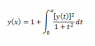# Solve for y(x) using the Fundamental Theorem of Calculus

• Kaylee

## Homework Statement

Solve the integral equation for y(x):
y(x) = 1 + ∫ { [y(t)]^2 / (1 + t^2) } dt
(integral from 0 to x)

See attached image for the equation in a nicer format.

## Homework Equations

Fundamental Theorem of Calculus

## The Attempt at a Solution

dy/dx = y(x)^2 / (1 + x^2)
∫ dy/y^2 = ∫ dx/(1 + x^2)
-1/y = arctan(x) + C
y = -1/arctan(x) + C

y(0) = 1 = -1/arctan(0) + C
C = 1 + 1/arctan(0)
Since arctan(0) = 0, the fraction is indeterminate.

In lectures we only did one practice example, so if anyone could give me a hand and point out where I am going wrong, it'll be greatly appreciated.

#### Attachments

•Calculus Equation.png
949 bytes · Views: 478

## Homework Statement

Solve the integral equation for y(x):
y(x) = 1 + ∫ { [y(t)]^2 / (1 + t^2) } dt
(integral from 0 to x)

See attached image for the equation in a nicer format.

## Homework Equations

Fundamental Theorem of Calculus

## The Attempt at a Solution

dy/dx = y(x)^2 / (1 + x^2)
∫ dy/y^2 = ∫ dx/(1 + x^2)
-1/y = arctan(x) + C
y = -1/arctan(x) + C

y(0) = 1 = -1/arctan(0) + C
C = 1 + 1/arctan(0)
Since arctan(0) = 0, the fraction is indeterminate.

In lectures we only did one practice example, so if anyone could give me a hand and point out where I am going wrong, it'll be greatly appreciated.

Use parentheses! -1/arctan(x)+C is not the same thing as -1/(arctan(x)+C)! One is indeterminant, one is -1/C. Which one do you want?

## Homework Statement

Solve the integral equation for y(x):
y(x) = 1 + ∫ { [y(t)]^2 / (1 + t^2) } dt
(integral from 0 to x)

See attached image for the equation in a nicer format.

## Homework Equations

Fundamental Theorem of Calculus

## The Attempt at a Solution

dy/dx = y(x)^2 / (1 + x^2)
∫ dy/y^2 = ∫ dx/(1 + x^2)
-1/y = arctan(x) + C
y = -1/(arctan(x) + C)

y(0) = 1 = -1/arctan(0) + C
C = 1 + 1/arctan(0)
Since arctan(0) = 0, the fraction is indeterminate.
This is a good example of why you need to not be sloppy about using parentheses.

: Dick wins again.

Use parentheses! -1/arctan(x)+C is not the same thing as -1/(arctan(x)+C)! One is indeterminant, one is -1/C. Which one do you want?
I definitely want -1/C. Thank you for helping me figure it out. I knew it was something small but I couldn't see it.

This is a good example of why you need to not be sloppy about using parentheses.

: Dick wins again.
It wasn't that I was sloppy. I commonly leave the C on the outer edge of my equations because C cannot loss its generality. But it definitely reminded me to use the generality to my advantage. Thank you to Dick and yourself for helping students with their problems.

It wasn't that I was sloppy. I commonly leave the C on the outer edge of my equations because C cannot loss its generality. But it definitely reminded me to use the generality to my advantage. Thank you to Dick and yourself for helping students with their problems.

Once you've put in the C after you integrate, you have to leave it where it is. You can't just move it someplace else later on without a good algebraic reason. It doesn't have that much 'generality'. -1/(arctan(x)+C) is a general solution to your ode. -1/arctan(x)+C is NOT.

Once you've put in the C after you integrate, you have to leave it where it is. You can't just move it someplace else later on without a good algebraic reason. It doesn't have that much 'generality'. -1/(arctan(x)+C) is a general solution to your ode. -1/arctan(x)+C is NOT.
Thanks. I didn't know that.